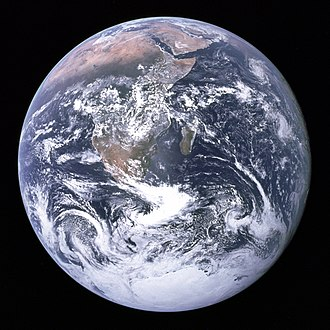### The secrets of the blue marble(Earth).

This article about knowing some of the known and unknown secrets of the earth and the measurements, orbital and physical characteristics of Earth.The Earth

## Are there any Alternative names for the earth?

• The answer is yes, there are some alternative names for the Earth they are Terra, Tellus, Gaia, Gaea, the World.
• And the Adjective form of the name of the Earth is Earthly, terrestrial, Terran, tellurian.

## The Orbital characteristics of the Earth

• The apsides of the Earth is Aphelion:152100000 km(94500000 mi; 1.017 AU), Perihelion:147095000 km(91401000 mi; 0.98327 AU).
• The Semi-major axis of Earth is 149598023 km(92955902 mi; 1.00000102 AU).
• The Eccentricity of the Earth is 0.0167086.
• The Orbital period of the Earth in 365.256363004 days(1.00001742096 yr).
• The Average orbital speed of the Earth is 29.78 km/s(107200 km/h; 66600 mph).
• The Mean anomaly of Earth is 358.617°.
• The Inclination of the Earth is 7.155° to the Sun's equator;1.57869° to invariable plane;0.00005° to J2000 ecliptic.
• The Longitude of ascending node of Earth is −11.26064° to J2000 ecliptic.
• The Argument of the perihelion of  Earth is 114.20783°.
• The satellities of the earth are

1. 1 natural satellite: the Moon
2. 5 quasi-satellites
3. 1 800 operational artificial satellites
4. 16 000 space debris

## The Physical Characteristics of the Earth

• The Mean radius of Earth is 6371.0 km (3958.8 mi).
• The Equatorial radius of Earth is 6378.1 km (3963.2 mi).
• The Polar radius of Earth is 6356.8 km (3949.9 mi).
• The Ellipticity of Earth is 0.0033528,1/298.257222101 (ETRS89).
• The Circumference of Eart is 40075.017 km equatorial (24901.461 mi),40007.86 km meridional (24859.73 mi).
• The total Surface area of Earth is 510072000 km2 (196940000 sq mi),148940000 km2 land (57510000 sq mi; 29.2%),361132000 km2 water (139434000 sq mi; 70.8%).
• The total Volume of Earth is1.08321×1012 km3 (2.59876×1011 cu mi).
• The total mass of the Earth is  5.97237×1024 kg (1.31668×1025 lb),(3.0×10−6 M☉).
• The Mean density of Earth is 5.514 g/cm3 (0.1992 lb/cu in).
• The Surface gravity of Earth is 9.80665 m/s2 (1 g; 32.1740 ft/s2).
• The Normalized polar Moment of the inertia factor of Earth is 0.3307.
• The Escape velocity of Earth is 11.186 km/s(40270 km/h; 25020 mph).
• The Sidereal rotation period of Earth is 0.99726968 days(23h 56m 4.100s).
• The Equatorial rotation velocity of Earth is 0.4651 km/s(1674.4 km/h; 1040.4 mph).
• The Axial tilt of Earth is 23.4392811°.
• The Albedo of Earth is 0.367 geometric,0.306 Bond.
• Surface temp.                 min               mean                                 max
• Kelvin:                            184 K      287.16 K (years 1961-1990)    330 K
• Celsius:                          −89.2 °C 14.0 °C (years 1961-1990) 56.9 °C
• Fahrenheit:                  −128.5 °F 57.2 °F (years 1961-1990) 134.3 °F

## The atmosphere of Earth consists of?

The surface pressure of Earth is 101.325 kPa (at MSL)

## Gases% in Earth

1. 78.08% nitrogen (N2; dry air)
2. 20.95% oxygen (O2)
3. 1% water vapor (climate variable)
4. 0.9340% argon
5. 0.0408% carbon dioxide
6. 0.00182% neon
7. 0.00052% helium
8. 0.00017% methane
9. 0.00011% krypton
10. 0.00006% hydrogen

So this is all about The secrets of the blue marble(Earth). Hope you like it.